## How to sumif adjacent cell is equal, blank or containing text in Excel?

While using Microsoft Excel, you may need to sum values where the adjacent cell equals a criterion in a certain range, or sum the values where the adjacent cell is blank or containing text. In this tutorial, we will provide you with formulas to deal with these problems.

Sumif adjacent cell equals a criterion in Excel
Sumif adjacent cell is blank in Excel
Sumif adjacent cell containing text in Excel

#### Sumif adjacent cell equals a criterion in Excel

As below screenshot shows, you need to sum all prices of the Beef. Please do as follows.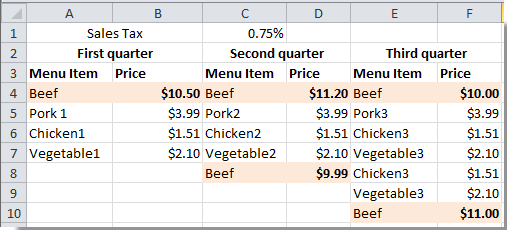1. Specify a cell for displaying the result.

2. Copy and paste the formula =SUM(IF(A4:E10=A13, B4:F10, 0)) into the Formula Bar, and then press Ctrl + Shift +Enter keys simultaneously to get the result.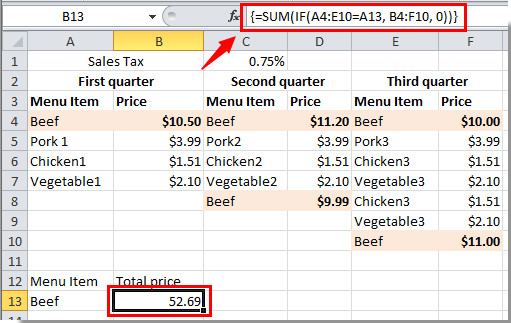Note: In the formula, A4:E10 is the data range which contains the specific criteria you need, A13 is the criteria that you want to sum the cells based on, and B4:F10 is the range which has the values you want to sum. . Please change the range based on your own data.

Easily combine duplicates in a column and sum values in another column based on the duplicates in Excel

The Kutools for Excel's Advanced Combine Rows utility helps you easily combine duplicate rows in a column and calculate or combine values in another column based on the duplicates in Excel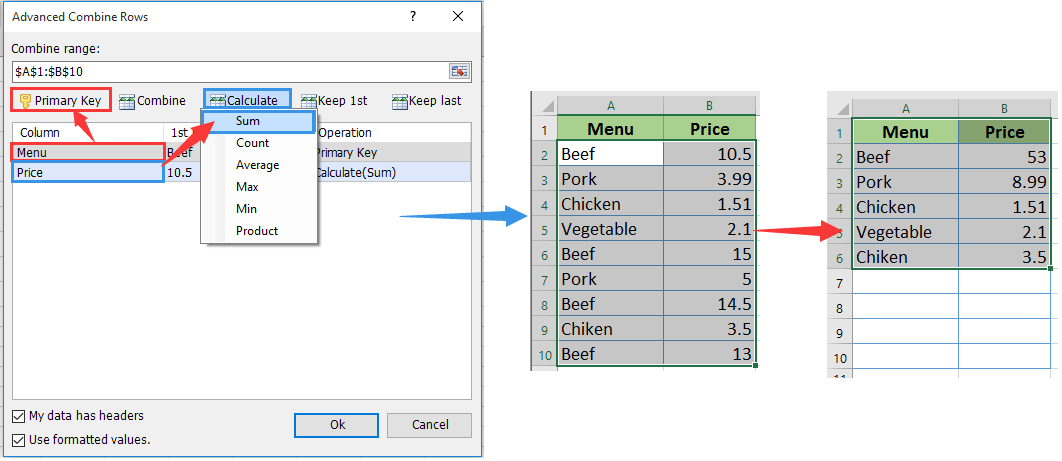#### Sumif adjacent cell is blank in Excel

For example, you have a range A2:B7 and you just need to sum the values where the adjacent cells are blank. See screenshot below. You need to do as follows.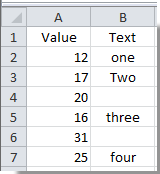1. Select a blank cell to display the result. Copy and paste the formula =SUM(IF(ISBLANK(B2:B7),A2:A7,0)) (B2:B7 is the data range which contains the blank cells, and A2:A7 is the data you want to sum) into the Formula Bar, then press Ctrl + Shift + Enter keys at the same time to get the result.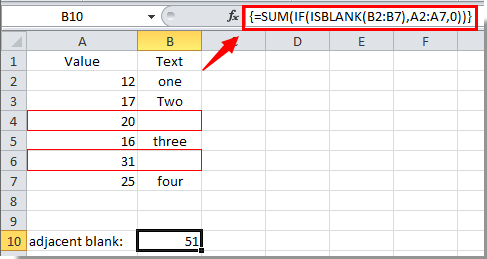Then you can see all values where the adjacent cells are blank are summed and displayed in the specified cell.

#### Sumif adjacent cell containing text in Excel

The above method helps you sum values where the adjacent cell is blank in a certain range. In this section, you will learn how to sum values where the adjacent cells contain texts in Excel.

Just use the same example as above method shown.

1. Select a blank cell, copy and paste the formula =SUMIF(B2:B7,"<>"&"",A2:A7) ( B2:B7 is the data range which contains the text cells, and A2:A7 is the data you want to sum) into the Formula Bar, then press Ctrl + Shift + Enter keys.

You will see all values where the adjacent cells contain texts are summed and displayed in the selected cell.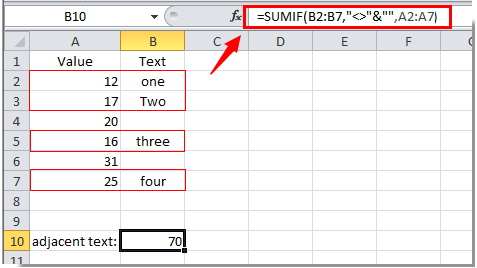### Best Office Productivity Tools

 Popular Features: Find, Highlight or Identify Duplicates   |  Delete Blank Rows   |  Combine Columns or Cells without Losing Data   |   Round without Formula ... Super Lookup: Multiple Criteria VLookup  |   Multiple Value VLookup  |   VLookup Across Multiple Sheets   |   Fuzzy Lookup .... Advanced Drop-down List: Quickly Create Drop Down List   |  Dependent Drop Down List   |  Multi-select Drop Down List .... Column Manager: Add a Specific Number of Columns  |  Move Columns  |  Toggle Visibility Status of Hidden Columns  |  Compare Ranges & Columns ... Featured Features: Grid Focus   |  Design View   |   Big Formula Bar   |  Workbook & Sheet Manager   |  Resource Library (Auto Text)   |  Date Picker   |  Combine Worksheets   |  Encrypt/Decrypt Cells   |  Send Emails by List   |  Super Filter   |   Special Filter (filter bold/italic/strikethrough...) ... Top 15 Toolsets:  12 Text Tools (Add Text, Remove Characters, ...)   |   50+ Chart Types (Gantt Chart, ...)   |   40+ Practical Formulas (Calculate age based on birthday, ...)   |   19 Insertion Tools (Insert QR Code, Insert Picture from Path, ...)   |   12 Conversion Tools (Numbers to Words, Currency Conversion, ...)   |   7 Merge & Split Tools (Advanced Combine Rows, Split Cells, ...)   |   ... and more

Supercharge Your Excel Skills with Kutools for Excel, and Experience Efficiency Like Never Before. Kutools for Excel Offers Over 300 Advanced Features to Boost Productivity and Save Time.  Click Here to Get The Feature You Need The Most...#### Office Tab Brings Tabbed interface to Office, and Make Your Work Much Easier

• Enable tabbed editing and reading in Word, Excel, PowerPoint, Publisher, Access, Visio and Project.
• Open and create multiple documents in new tabs of the same window, rather than in new windows.
• Increases your productivity by 50%, and reduces hundreds of mouse clicks for you every day!No ratings yet. Be the first to rate!
This comment was minimized by the moderator on the site
Sumif Adjacent Cell Equals A Criterion In Excel formula is not working for me, it is adding everything instead of just the cells with data we want.
This comment was minimized by the moderator on the site
Hi Cole,
Which Excel version are you using? Would you mind providing a screenshot of your data?
This comment was minimized by the moderator on the site
Hello I am trying to set up a pricing list. I have used a dropdown list for the selected products. How do I make excel input a price based on the choice of the text in the dropdown list.
This comment was minimized by the moderator on the site
Hi erin,
This comment was minimized by the moderator on the site
I am trying to get a return value of "yes" if A1:A7 contains any text. What formulas can I use?
This comment was minimized by the moderator on the site
Dear Wyman Thomas,
Supposing you need to return value of "Yes" if cell A1 contains "Apple", this formula can help you =IF(ISNUMBER(SEARCH("Apple",A1)), "Yes", "No").
This comment was minimized by the moderator on the site
Pretty sure

=SUMIF(B2:B7,"<>"&"",A2:B7) should be

=SUMIF(B2:B7,"<>"&"",A2:A7)
This comment was minimized by the moderator on the site
Few People Notice that, We take it A2:A7
This comment was minimized by the moderator on the site
Hi,
This comment was minimized by the moderator on the site
Dear all. I would like to use the SUMIF function in the following way: -Sum range is 1:1 -Criteria Range is 2:2 -Criteria is "jorge" However, I would like the function o work a little bit different. If "jorge" is found on B2, I would like A1 to be sum instead of A2. I tried =SUMIF(1:1,OFFSET(2:2,,1),"jorge") but excel does not accept such criteria range argument. How to get it working?
This comment was minimized by the moderator on the site
I am looking to create a budget spreadsheet. My goal is to plug in my daily expenses, with the category in one cell and the amount in the cell beneath. As far as my spending goes, I have no problem calculating. However, I am struggling with a formula to create a running tally of each category. Can you help?
This comment was minimized by the moderator on the site
Mike I am working on the same problem I created a drop down list for column D and my costs are in column C. Simply change the name (Contracts) of the formula to your category name. =SUMIF(D2:D31,""Contracts"",C2:C31)
This comment was minimized by the moderator on the site
hi, hope you could help me on this i want to create a formula where-in the codes under the subnet, be reflected on the net. see below place(net) 1 place1 2 place2 3 place3 4 place4 5 place5 where place net should have a code 1+2+3+4+5, i know how to concatenate and use "&" but i need to have a formula where i could drag it down since its not the only net in my specs, hoping for your answer? bog thanks regards, jayson
This comment was minimized by the moderator on the site
I would like to get the return value "yes" if the cells in A1:A7 contain data. What formula can I use?
There are no comments posted here yet